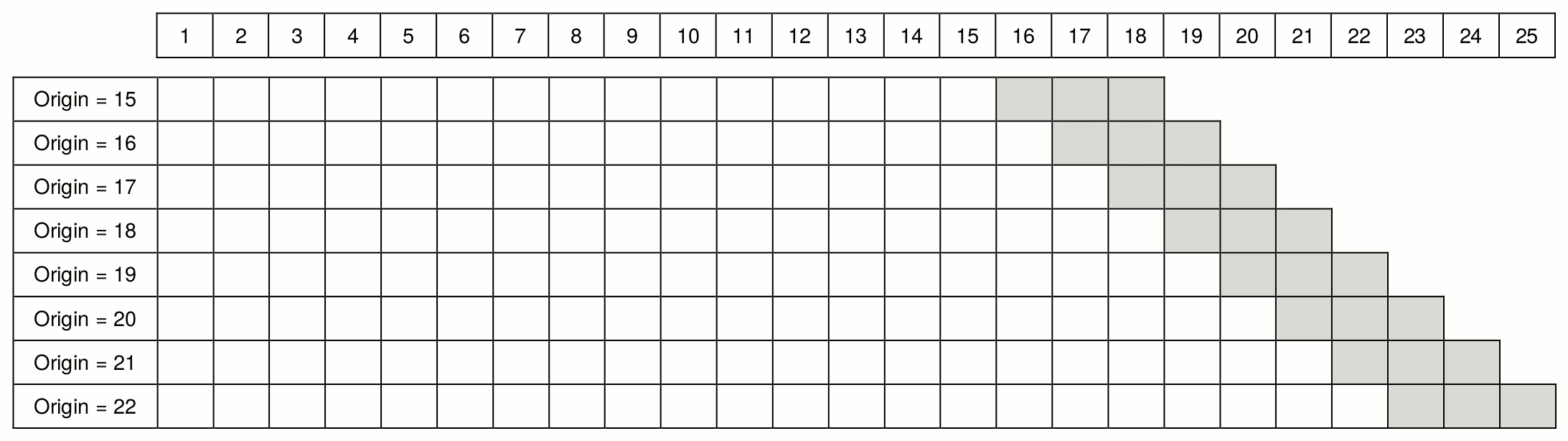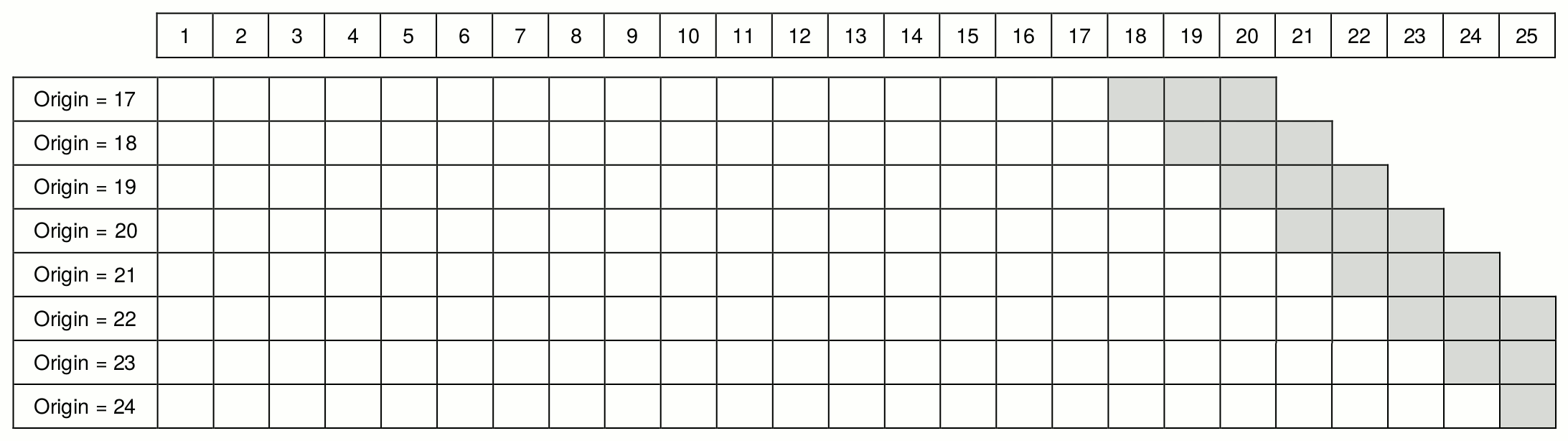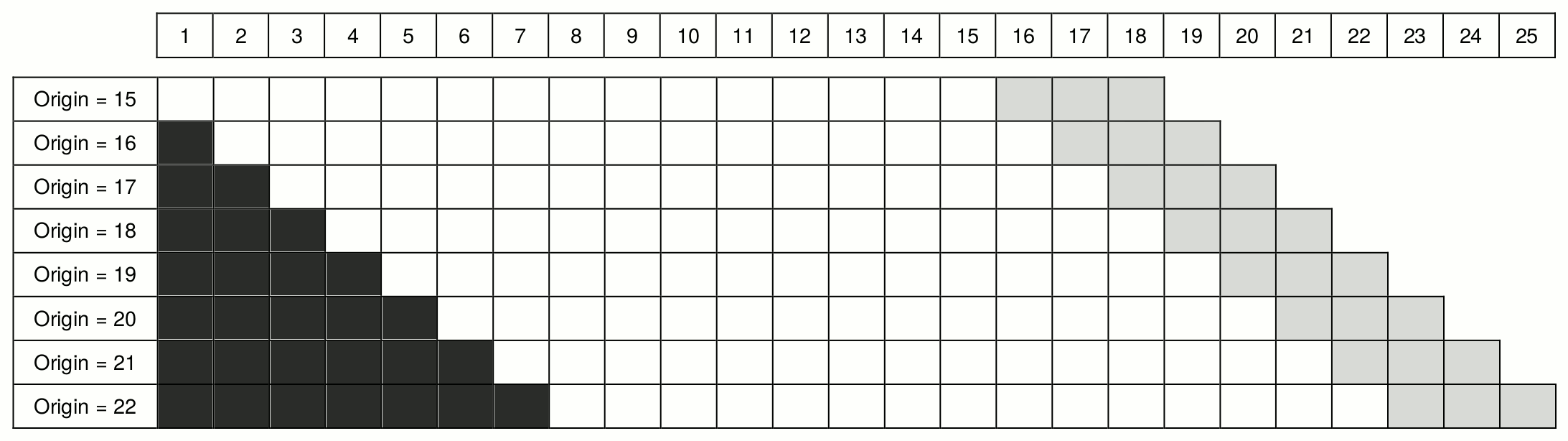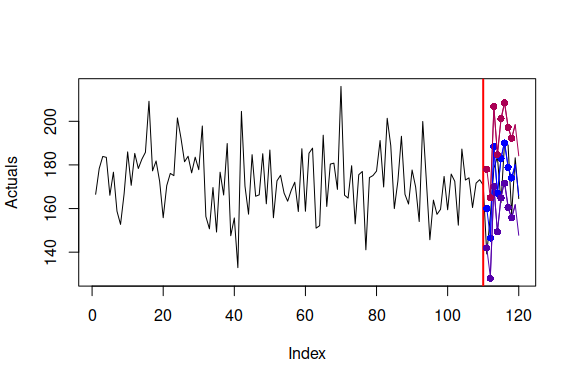# Rolling Origin

#### 2021-09-21

When there is a need to select the most appropriate forecasting model or method for the data, the forecasters usually split the available sample into two parts: in-sample (aka “training set”) and holdout sample (or out-sample, or “test set”). The model is then estimated on in-sample and its forecasting performance is evaluated using some error measure on the holdout sample.

If such a procedure done only once, then this is called “fixed origin” evaluation. However, the time series might contain outliers or level shifts and a poor model might perform better than the more appropriate one only because of that. In order to robustify the evaluation of models, something called “rolling origin” is used.

Rolling origin is an evaluation technique according to which the forecasting origin is updated successively and the forecasts are produced from each origin (Tashman 2000). This technique allows obtaining several forecast errors for time series, which gives a better understanding of how the models perform. There are different options of how this can be done.

## How can this be done?

The figure below (from (Svetunkov and Petropoulos 2018)) depicts the basic idea of rolling origin. White cells correspond to the in-sample data, while the light grey cells correspond to the three-steps-ahead forecasts. Time series has 25 observations in that figure, and the forecasts are produced from 8 origins, starting from the origin 15. The model is re-estimated on each iteration, and the forecasts are produced. After that a new observation is added at the end of the series and the procedure continues. The process stops when there is no more data to add. This could be considered as a rolling origin with a constant holdout sample size. As a result of this procedure 8 one to three steps ahead forecasts are produced. Based on them we can calculate the preferred error measures and choose the best performing model.Another option of producing forecasts from 8 origins would be to start from the origin 17 instead of 15 (see Figure below). In this case the procedure continues until origin 22, when the last three-steps-ahead forecast is produced, and then continues with the decreasing forecasting horizon. So the two-steps-ahead forecast is produced from the origin 23 and only one-step-ahead forecast is produced from the origin 24. As a result we obtain 8 one-step-ahead forecasts, 7 two-steps-ahead forecasts and 6 three-steps-ahead forecasts. This can be considered as a rolling origin with a non-constant holdout sample size. This can be useful in cases of small samples, when we don’t have any observations to spare.Finally, in both of the cases above we had the increasing in-sample size. However for some research purposes we might need a constant in-sample. The figure below demonstrates such a situation. In this case on each iteration we add an observation at the end of the series and remove one from the beginning of the series (dark grey cells).## What does this have to do with R?

The function ro() from greybox package (written by Yves Sagaert and Ivan Svetunkov in 2016 on the way to the International Symposium on Forecasting) implements the rolling origin evaluation for any function you like with a predefined call and returns the desired value. It heavily relies on the two variables: call and value - so it is quite important to understand how to formulate them in order to get the desired results. Overall, ro() is a very flexible function, but, as a result, it is not very simple. Let’s see how it works.

x <- rnorm(100,100,10)

We use ARIMA(0,1,1) for this example:

ourCall <- "predict(arima(x=data,order=c(0,1,1)),n.ahead=h)"

The call that we specify includes two important elements: data and h. data specifies where the in-sample values are located in the function that we want to use, and it needs to be called “data” in the call. h will tell our function, where the forecasting horizon is specified in the selected function. Note that in this example we use arima(x=data,order=c(0,1,1)), which produces a desired ARIMA(0,1,1) model and then we use predict(...,n.ahead=h), which produces a forecast from that model. The part arima(x=data,order=c(0,1,1)) can also be simplified to arima(data,order=c(0,1,1)) according to the general rules of R.

Having the call, we need also to specify what the function should return. This can be the conditional mean (point forecasts), prediction intervals, the parameters of a model, or, in fact, anything that the model returns (e.g. name of the fitted model and its likelihood). However, there are some differences in what the ro() returns depending on what the function you use returns. If it is a vector, then ro() will produce a matrix (with values for each origin in columns). If it is a matrix, then an array is returned. Finally, if it is a list, then a list of lists is returned.

In order not to overcomplicate things, let’s start with collecting the conditional mean from the predict() function:

ourValue <- "pred"

NOTE: If you do not specify the value to return, the function will try to return everything, but it might fail, especially if a lot of values are returned. So, in order to be on the safe side, always provide the value, when possible.

Now that we have specified ourCall and ourValue, we can produce forecasts from the model using rolling origin. Let’s say that we want three-steps-ahead forecasts and 8 origins with the default values of all the other parameters:

returnedValues1 <- ro(x, h=3, origins=8, call=ourCall, value=ourValue)

The function returns a list with all the values that we asked for plus the actual values from the holdout sample. We can calculate some basic error measure based on those values, for example, scaled Mean Absolute Error (Petropoulos and Kourentzes 2015):

}
ourHoldoutValues[j,,] <- ourROReturn$holdout } Although we do not specify i explicitly anywhere in the call of ro() above, it is used in ourCall and as a result the different models will be estimated in the inner loop. Comparing the performance of the two on the different time series we have (this is RelMAE from (Davydenko and Fildes 2013)): exp(mean(log(apply(abs(ourHoldoutValues - ourForecasts[,1,,]),1,mean,na.rm=TRUE) / apply(abs(ourHoldoutValues - ourForecasts[,2,,]),1,mean,na.rm=TRUE)))) #>  0.7365528 So based on these results, it can be concluded, that ARIMA(0,1,1) is on average more accurate than ARIMA(1,1,0) on our three time series. ## Making things even more complicated exciting For our last examples we create the data frame and try fitting linear regression: xreg <- matrix(rnorm(120*3,c(100,50,150),c(10,5,15)), 120, 3, byrow=TRUE) y <- 0.5*xreg[,1] + 0.2*xreg[,2] + 0.75*xreg[,3] + rnorm(120,0,10) xreg <- cbind(y,xreg) colnames(xreg) <- c("y",paste0("x",c(1:3))) xreg <- as.data.frame(xreg) Note that in this example we cheat ro() function and make a call, that does not contain either data or h, because the regression implemented in lm() function relies on data frames and does not use forecasting horizon: ourCall <- "predict(lm(y~x1+x2+x3,xreg[counti,]),newdata=xreg[counto,],interval='p')" In this case we just need to make sure that the Global Environment contains the xreg data frame. counti variable in the call is needed for the internal loop - it determines the length of the in-sample. Similarly counto specifies the length of the holdout. Both of them are defined inside the ro() function. In addition, we don’t need to specify ourValue, because the function predict.lm() returns a matrix with values (or a vector if we don’t ask for intervals), not a list. NOTE: if you use a different function (not lm()), then you might need to specify the value. The final call to ro() is as usual pretty simple: ourROReturn <- ro(xreg$y, h=3, origins=8, call=ourCall, ci=TRUE, co=TRUE)
#> Warning: You have not specified the 'value' to produce.We will try to return
#> everything, but we cannot promise anything.

In this case, we need to provide the response variable in the data parameter of the call, because the function needs to extract values for the holdout.

Similar thing can be done using alm() function but with a proper value (the function might fail otherwise):

ourCall <- "predict(alm(y~x1+x2+x3,xreg[counti,]),newdata=xreg[counto,],interval='p')"
ourValue <- c("mean","lower","upper")
ourROReturn <- ro(xreg\$y, h=3, origins=8, call=ourCall, value=ourValue, ci=TRUE, co=TRUE)
plot(ourROReturn)As a final example, we consider ARIMAX model for the following data:

xreg <- matrix(rnorm(120*3,c(100,50,150),c(10,5,15)), 120, 3, byrow=TRUE)
y <- 0.5*xreg[,1] + 0.2*xreg[,2] + 0.75*xreg[,3] + rnorm(120,0,10)
colnames(xreg) <- paste0("x",c(1:3))
xreg <- as.data.frame(xreg)

and modify the call accordingly:

ourCall <- "predict(arima(x=data, order=c(0,1,1), xreg=xreg[counti,]), n.ahead=h, newxreg=xreg[counto,])"

Taking that now we deal with ARIMA, we need to specify both data and h. Furthermore, xreg is different than in the previous example, as it now should not contain the response variable.

As usual, we need our function to return the specific predicted values:

ourValue <- "pred"

And then we call for the function and get the results:

ourROReturn <- ro(x, h=3, origins=8, call=ourCall, value=ourValue)

As a side note, if you use smooth package for R and want to do rolling origin evaluation, for example, for ETSX model using es() function, the call can be simplified to:

ourCall <- "es(x=data, xreg=xreg[countf,]), h=h)"

Here we use countf variable, which specifies that we need to use both in-sample and the holdout (the full sample), as the function es() does not rely on forecast() or predict() and can produce forecasts internally.

Finally, all of the examples mentioned above can be done in parallel, which is triggered by parallel parameter. Note that this might be useful for cases, when number of origins is very high and the sample size is large. Otherwise this might be less efficient than the serial calculation. If you want to do anything in parallel, then you either need either doMC (Linux and Mac OS) or doParallel (for Windows) package in R. The number of cores used in this case is equal to the number of cores of your CPU minus one.